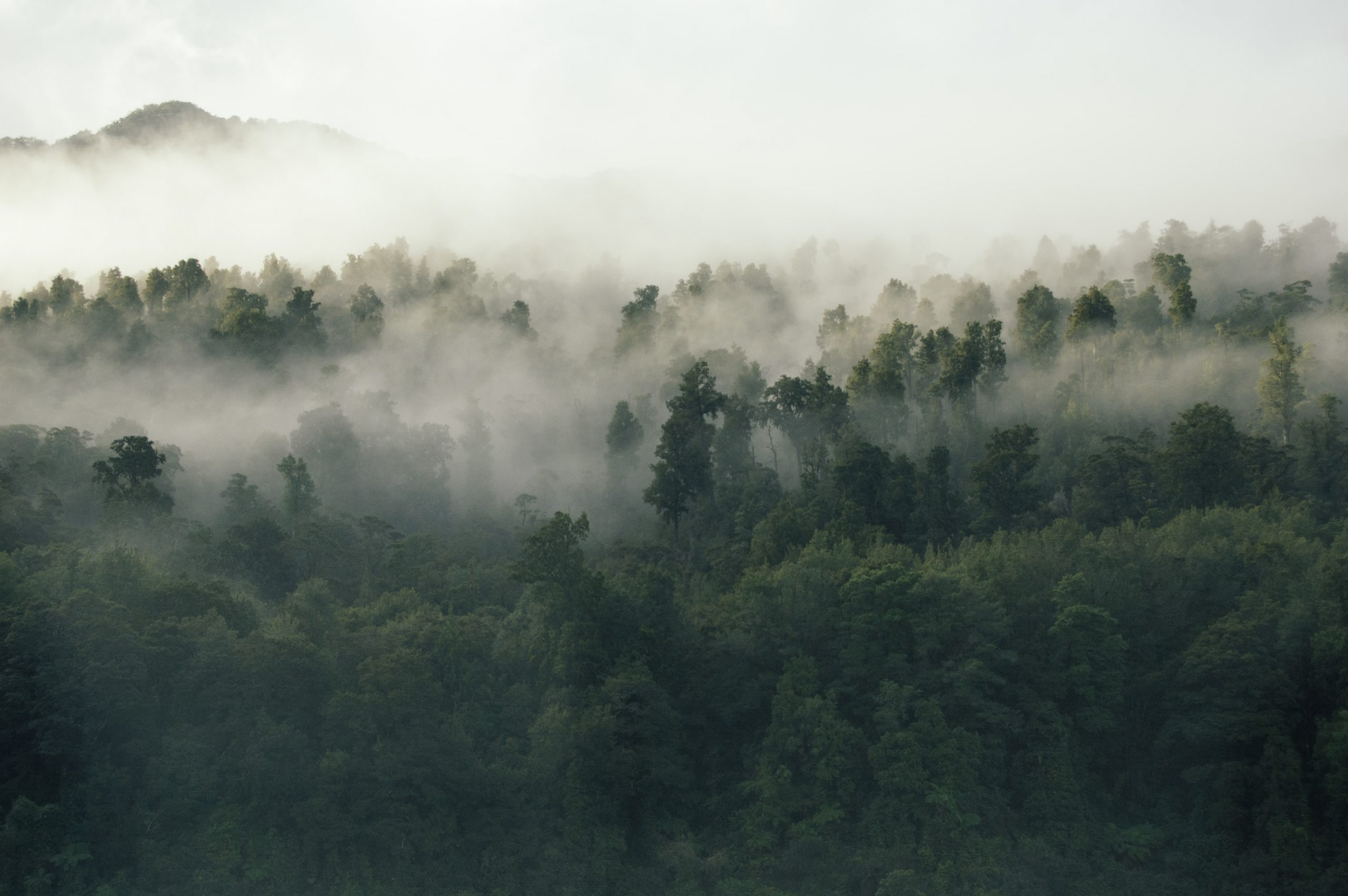## Measure Carbon Sequestration from a Tree

June 4, 2021How to measure carbon from a tree?

Once a tree or plant is planted, that tree grows. As it grows, its stems and leaves also grow. In the same way, the roots also grow. As a tree grows, so does the amount of carbon it stores. As long as the tree continues to grow, the carbon reserves will also increase. The International Panel on Climate Change(IPCC) has provided guidance on how to measure carbon in the world. But if the formulas for the method of measuring the trees after preparing an allometric equation according to the climate of the country are worked out, its universality will be more. But so far, no allometric equation has been prepared in Nepal.

If someone has planted a tree and is growing it, it is possible to measure how much carbon has been conserved and how much contributed to reducing the emissions into the atmosphere by measuring carbon in the following ways.

Measurement of a tree height and diameter of a tree

The initial measure of how much carbon a tree stores is to measure that tree. For measuring, the height of the trunk should be taken in meters and the diameter of the stem taken at the height of 1.3 meters of the tree, i.e. usually taken from the height of the chest.

The height of the tree is usually taken by an instrument called a suntometer or a hypsometer, turning from the base of the tree to the plane at an angle with the top of the tree as the focal point. The angle of view at the top of the tree should be 45 degrees by walking back and forth. If that angle is 45 degrees, the height of the tree is calculated by adding the distance from the base of the tree to the place where the height is to be measured and the height of the person measuring the tree.

A.   Carbon Calculation of a tree

As the trees grow, so does the carbon they store. But the order and extent of its growth depends on the climate and growth rate of each species. According to the nature of the trees, the carbon reserves go up and down. The simplest way is to estimate how much carbon a tree can store. These methods may not apply everywhere. This may not be 100% accurate, but it does give you a closer look.

How much carbon is stored in a tree can be calculated as follows.

Step 1: Determine the total green weight of the tree

The green weight is the weight of the tree when it is alive. First, you have to calculate the green weight of the above-ground weight as follows:

Wabove-ground= 0.25 D2 H (for trees with D<11)

Wabove-ground= 0.15 D2 H (for trees with D>11)

Wabove-ground= Above-ground weight in pounds x 0.45= …….Kg

D = Diameter of the trunk in inches

H = Height of the tree in feet

The root system weight is about 20% of the above-ground weight. Therefore, to determine the total green weight of the tree, multiply the above-ground weight by 1.2:

Wtotal green weight = 1.2* Wabove-ground

Step 2: Determine the dry weight of the tree

The average tree is 72.5% dry matter and 27.5% moisture. Therefore, to determine the dry weight of the tree, multiply the total green weight of the tree by 72.5%.

Wdry weight = 0.725 * Wtotal green weight

Step 3: Determine the weight of carbon in the tree

The average carbon content is generally 50% of the tree’s dry weight total volume. Therefore, in determining the weight of carbon in the tree, multiply the dry weight of the tree by 50%.

Wcarbon = 0.5 * Wdry weight

Step 4: Determine the weight of carbon dioxide sequestered in the tree

CO2 has one molecule of Carbon and 2 molecules of Oxygen. The atomic weight of Carbon is 12 (u) and the atomic weight of Oxygen is 16 (u). The weight of CO2 in trees is determined by the ratio of CO2 to C is 44/12 = 3.67. Therefore, to determine the weight of carbon dioxide sequestered in the tree, multiply the weight of carbon in the tree by 3.67.

Wcarbon-dioxide = 3.67 * Wcarbon

Example of CO2 calculation

Tree details:

10 years old tree

5 meter tall or 16.4 feet tall (“H”)

25 cm trunk or 9.8 inch trunk (“D”)

Wabove-ground= 0.25 D2 H= 0.25(9.82)(16.4) = 394 x0.45= 177.3 Kg

Wtotal green weight = 1.2* Wabove-ground= 1.2 * 177.3 = 212.76 Kg

Wdry weight = 0.725 * Wtotal green weight= 0.725 * 212.76 = 154.25 Kg

Wcarbon = 0.5 * Wdry weight  = 0.5 * 154.25 = 77.12 Kg

Wcarbon-dioxide = 3.67 * Wcarbon  = 3.67 * 77.12 = 283.03 Kg CO2 sequestered in 10 years;

Ultimately, the growth of each tree is non-linear, and the greatest sequestration stage is in the younger stages of tree growth, depending on rates and peaks of individual species, with the sequestration of CO2 per year dropping thereafter.

CO2 sequestration can differ even within tree species, with multiple factors such as growth conditions also at play. But while the exact CO2 sequestration rates may require more accurate measurements to pinpoint, the impact trees can create is undeniable in our global fight against climate change, in addition to the host of localized functions it can fulfill.

In the forest land, soil carbon is also measured therefore please consult further references for soil carbon measurement.

References

How to calculate C02 Sequestration
https://www.ecomatcher.com/how-to-calculate-co2-sequestration/